# Convergence results for fixed point iterative algorithms in metric spaces

### Abstractcarpathian_2019_35_2_209_220_abstract

Let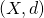be a metric space,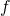,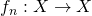, with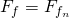,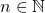. For the fixed point equation

(1)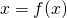we consider the following iterative algorithm,

(2)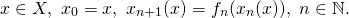By definition, the algorithm \eqref{equ2} is convergent if,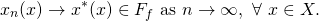In this paper we give some conditions on \underline{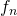and} which imply the convergence of algorithm \eqref{equ2}. In this way we improve some results given in [ Rus, I. A., {\em An abstract point of view on iterative approximation of fixed points: impact on the theory of fixed point equations}, Fixed Point Theory, \textbf{13} (2012), No. 1, 179–192].
In our results, in general we do not suppose that,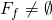. Some research directions are formulated.